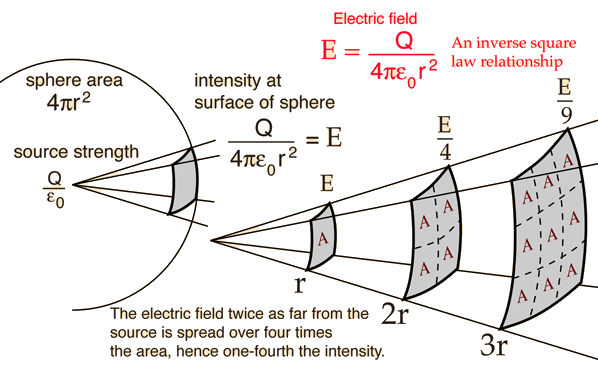# Topic 10: Fields (HL)

See the guide for this topic.

## 10.1 – Describing fields

• ### Gravitational fields

A gravitational field is a space where a small test mass experiences a force due to another mass.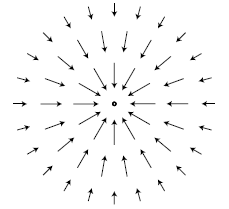• ### Electrostatic fields

An electrostatic field is a space where a small positive test charge experiences a force per unit charge.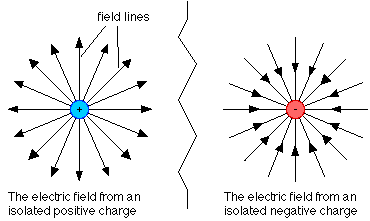• ### Electric potential and gravitational potential

Electric potential

• The electric potential is given byor• The electric potential difference is also known as voltage.

See section 10.2 (potential difference) for further explanation.

Gravitational potential

• The gravitational potential due to an object with mass M is given by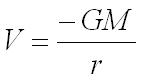• The gravitational potential at a point P is equal to work done per unit mass required to take a test mass from infinity to point P.
• Gravitational potential is always negative.
• The gravitational potential at infinity is zero.
• To calculate the gravitational potential due to multiple masses, simply add up the gravitational potential due to the individual masses.

• ### Field lines

See next section (equipotential surfaces)

• ### Equipotential surfaces

• Points with the same gravitational potential can be joined together to form an equipotential surface• Field lines are normal (perpendicular) to the equipotential surfaces.• The density of field lines is proportional to the field strength.
• Far from the earth, the field line separation changes as the gravitational field strength changes. However, near the surface of the earth, the value of the gravitational field strength is relatively constant with height as long as the change of height is not too great.## 10.2 – Fields at work

• ### Potential and potential energy

Electric potential

See previous section (electric potential and gravitational potential)

Electric potential energy

• The electric potential energy is given by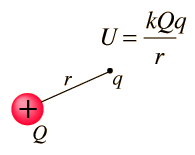were k is Coulomb’s constant, Q is the fixed charge, q is the test charge, and r is the radius.

• The electric potential energy can be defined as the capacity for doing work by a change in position of the positive test charge.
• Electric potential is also known as voltage.

Gravitational potential

See previous section (electric potential and gravitational potential)

Gravitational potential energy

• The gravitational potential energy of a system of two objects with mass M and m is given by• The gravitational potential energy of an object at a point P is equal to the work done required to take the object from infinity to the point P.
• As gravitational forces are attractive, the work done required to bring an object from infinity to any point is negative. Thus, gravitational potential energy is always negative.

• ### Potential gradient

• The gravitational potential gradient of a gravitational field is given by ΔV/Δr where ΔV is the change in gravitational potential between two points and Δr is the distance between those two points.
• It is the slope of a graph which plots the gravitational potential against the distance from the mass.
• Gravitational potential gradient is related to the gravitational field strength (g) by g=-ΔV/Δr=GM/r^2.

• ### Potential difference

• The potential difference is defined as the work done by moving a positive test charge from one point to another in an electric field.
• Potential difference across an electrical component, also called voltage, is required to make a current flow through it. Cells or batteries provide the potential difference required.• ### Escape speed

• The escape speed of a planet is given bywhere G is the gravitational constant, M is the mass of the planet, and R is the radius of the planet.

• Escape speed it the minimum speed required for an object launched from the surface of a planet to reach infinity.
• An object launched at or above its escape speed will not return to the planet due to gravity.• ### Orbital motion, orbital speed and orbital energy

Orbital motion

• Gravitation provides the centripetal force for orbital motion. For example, the gravitational force between the sun and the earth provides the centripetal force for the earth to orbit the sun.
• The period of orbital motion is proportional to the average radius of the orbital motion by Kepler’s third law.Orbital speed

• The orbital speed is given by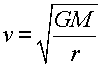where G is the gravitational constant, M is the mass of the planet, and r is the radius of the planet.

Orbital energy

The kinetic energy, gravitational potential energy, and total energy of an orbiting satellite is given by

• Kinetic energy• Potential energy• Total energy = Kinetic energy + Gravitational potential energy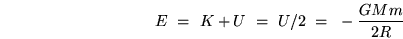• ### Forces and inverse-square law behaviour

• Inverse-square law graphical representation• Gravitational field• Electric field